Technical Article

# Learn about the Overlap-Add Method: Linear Filtering Based on the Discrete Fourier Transform

October 25, 2017 by Dr. Steve Arar

## The overlap-add method allows us to use the DFT-based method when calculating the convolution of very long sequences.

The overlap-add method allows us to use the DFT-based method when calculating the convolution of very long sequences.

In the first part of this series, we discussed the DFT-based method to calculate the time-domain convolution of two finite-duration signals. In practice, we generally need to calculate the convolution of very long sequences. We may even need to apply a Finite Impulse Response (FIR) filter on a real-time input sequence. In these cases, it is necessary to break the input sequence into finite-duration signals of an appropriate length.

There are two methods to perform DFT-based linear filtering on long sequences: overlap-add method and overlap-save method. In this article, we will review the overlap-add method. Then, we will compare the computational complexity of an FIR filter based on the DFT method with that of the direct-form realization.

We will explain this method using an example. Assume that $$x(n)$$ and $$h(n)$$ are as shown in Figure 1 and 2, respectively.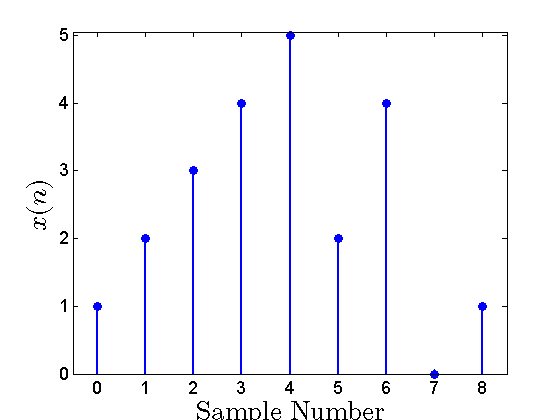##### Figure 1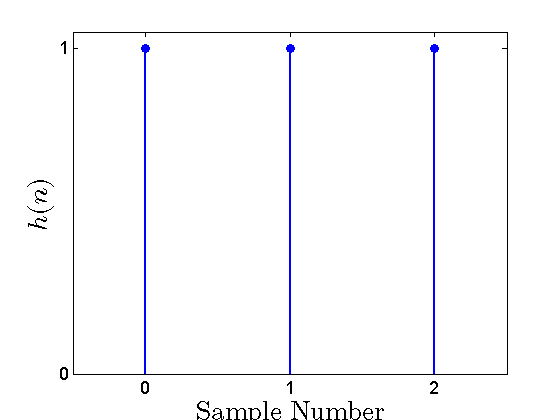##### Figure 2

We want to calculate the convolution of these two signals

$$y(n)=x(n) \star h(n)$$

##### Equation 1

$$x(n)$$ and $$h(n)$$ are not long sequences here and we can directly apply the DFT-based method to calculate their convolution; however, we will break $$x(n)$$ into three signals of length three to explain the concept of the overlap-add method. We can write

$$x(n)=x_{0}(n)+x_{1}(n-3)+x_{2}(n-6)$$

##### Equation 2

where $$x_0(n)$$, $$x_1(n)$$, and $$x_2(n)$$ are as shown in Figure 3, 4, and 5, respectively.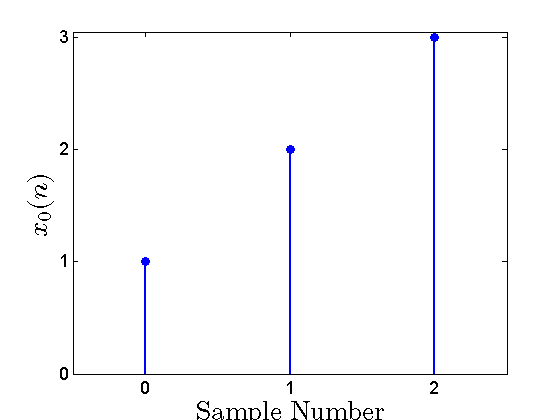##### Figure 3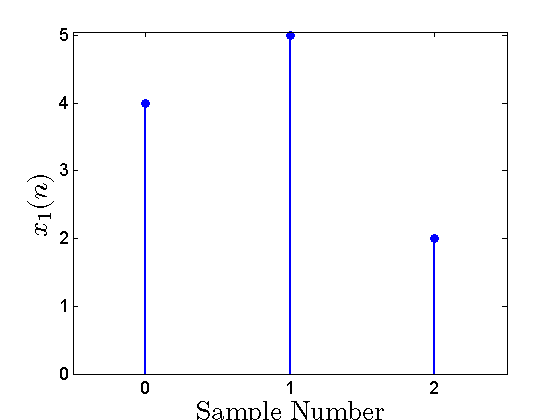##### Figure 4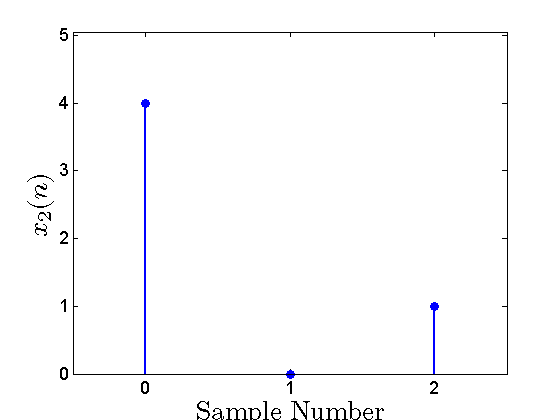##### Figure 5

Substituting Equation 2 into Equation 1 gives

$$y(n)=\big( x_{0}(n)+x_{1}(n-3)+x_{2}(n-6) \big) \star h(n)$$

The distributive property of the convolution gives

$$y(n)= x_{0}(n) \star h(n)+x_{1}(n-3) \star h(n)+x_{2}(n-6) \star h(n)$$

##### Equation 3

Each of the three terms on the right-hand side of the above equation can be considered as the output of a linear time-invariant system with the impulse response $$h(n)$$. Due to the time-invariant property, a time-shift of the input sequence leads to the same time-shift in the system’s output sequence. For example, to calculate $$x_1(n-3) \star h(n)$$, we can calculate $$x_1(n) \star h(n)$$ and, then, shift the result by three samples. Hence, defining

$$y_{0}(n)= x_{0}(n) \star h(n)$$

$$y_{1}(n)= x_{1}(n) \star h(n)$$

$$y_{2}(n)= x_{2}(n) \star h(n)$$

##### Equation 4

we can rewrite Equation 3 as

$$y(n)=y_{0}(n)+y_{1}(n-3)+y_{2}(n-6)$$

##### Equation 5

Now, the DFT-based method can be used to calculate the convolutions of Equation 4 which are of the desired length. Then, we can apply an appropriate time shift and calculate $$y(n)$$ as given by Equation 5. Since each of the $$x_0(n)$$, $$x_1(n)$$, and $$x_2(n)$$ are of length $$L=3$$ and $$h(n)$$ is of length $$K=3$$, we need DFTs longer than $$L+K-1=3+3-1=5$$ (see the previous article in this series). Since we only want to discuss the concepts, we won’t get involved in the mathematics. Using the MATLAB code given below, we can apply the DFT-based method to the convolutions of Equation 4.

x0=[1 2 3 0 0]; %This line defines the zero-padded version of x0(n)

h=[1 1 1 0 0]; %This line defines the zero-padded version of h(n)

ifft(fft(x0).*fft(h)); % This line calculates the IDFT of the product of the DFTs

The result will give $$y_0(n)$$ as:

1.0000    3.0000    6.0000    5.0000    3.0000

Similarly, we can calculate $$y_1(n)$$ and $$y_2(n)$$. These three signals are shown in Figure 6, 7, and 8.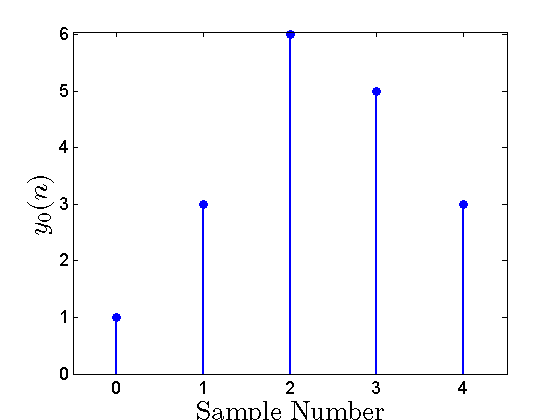##### Figure 6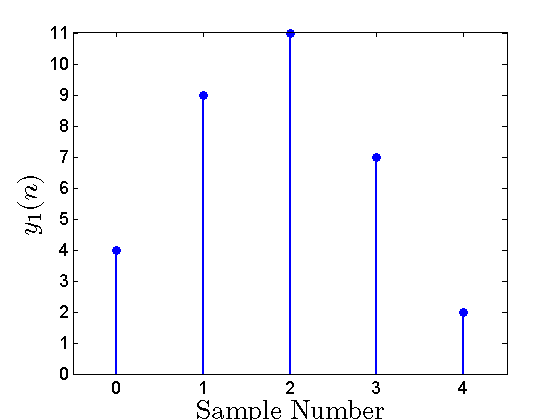##### Figure 7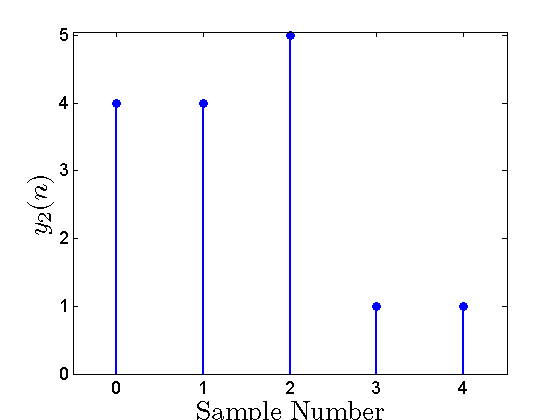##### Figure 8

Now, we only need to apply the appropriate time shift to $$y_1(n)$$ and $$y_2(n)$$ and then calculate $$y(n)$$ given by Equation 5. To better visualize the process, the shifted versions of $$y_0(n)$$, $$y_1(n)$$, and $$y_2(n)$$ are plotted in Figure 9.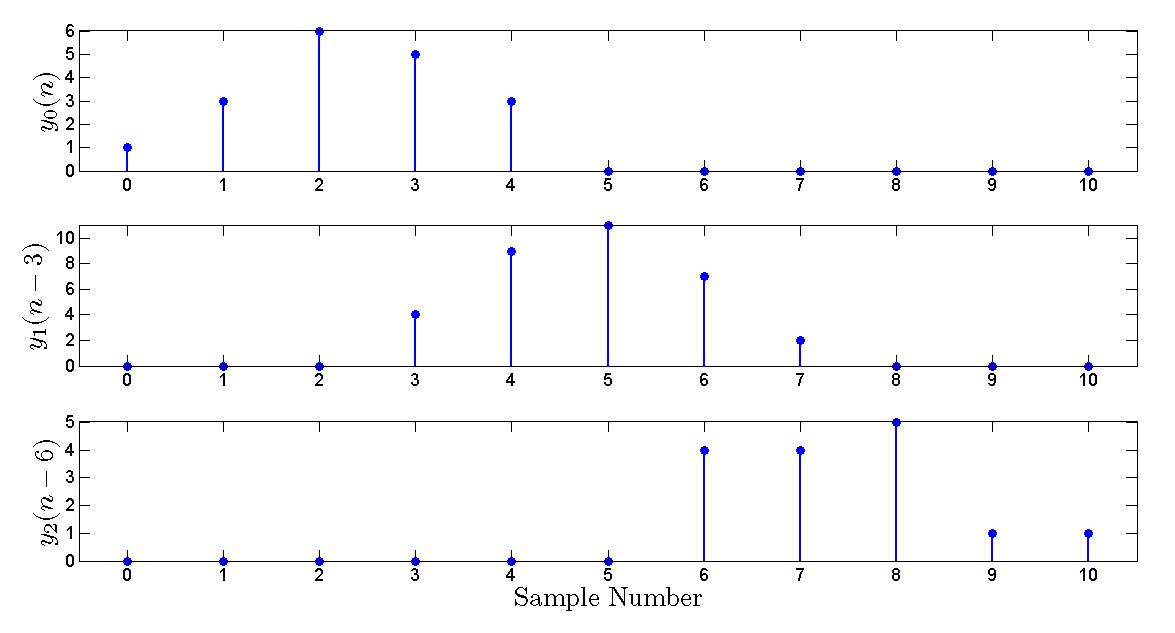##### Figure 9

Adding these signals together, we obtain $$y(n)$$ shown in Figure 10. Since, we are adding the samples that have overlap with each other, the discussed DFT-based convolution is referred to as the overlap-add method. To verify the result, we can use the MATLAB conv() function with the original $$x(n)$$ and $$h(n)$$ signals:

x=[1 2 3 4 5 2 4 0 1];

h=[1 1 1];

conv(x, h)

The result will be

1     3     6     9    12    11    11     6     5     1     1

which matches the DFT-based method.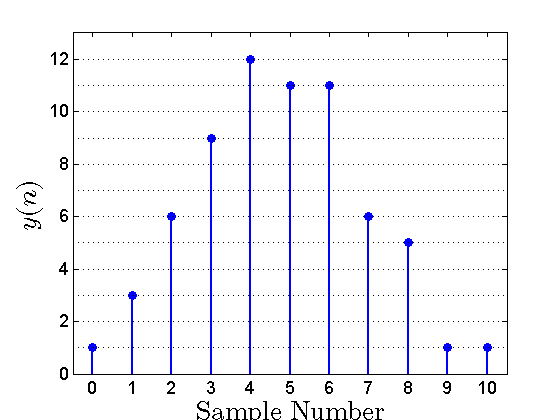##### Figure 10

Here’s the summary of our discussion so far: assume that we want to calculate the convolution of a long sequence, $$x(n)$$, with $$h(n)$$ of length $$K$$. To apply the overlap-add method, we should:

1- Break the long sequence,$$x(n)$$ , into signals of length $$L$$. If we specify the first $$L$$ samples of $$x(n)$$ as $$x_0(n)$$, the second $$L$$ samples as $$x_1(n)$$, and so on, we can write

$$x_{m}(n)= \begin{cases}x(n+mL) & 0\leq n \leq L-1 \\0 & otherwise\end{cases}$$

and clearly, we have

$$x(n)=x_{0}(n)+x_{1}(n-L)+x_{2}(n-2L)+\dots$$

2- Use the DFT-based method to calculate the convolution of each $$x_m(n)$$ with $$h(n)$$. Since $$x_m(n)$$ and $$h(n)$$ are of length $$L$$ and $$K$$, respectively, we need DFTs and inverse DFTs longer than $$N=L+K-1$$. Under this condition, the inverse DFT of the product of the DFTs will give the linear convolution and we will obtain

$$y_{m}(n)=x_{m}(n) \star h(n)$$

3- Shift each $$y_m(n)$$ by $$mL$$ samples and add the results together. Note that, in step 1, we divided $$x(n)$$ into blocks of length $$L$$ which span from sample index $$0$$ to $$L-1$$. Since the relative position of the blocks is not considered when calculating $$y_m(n)$$, we need to shift $$y_m(n)$$ by $$mL$$ samples and then add them together. Mathematically expressed, the final convolution will be

$$y(n)=y_{0}(n)+y_{1}(n-L)+y_{2}(n-2L)+ \dots$$

### Computational Complexity

Let’s consider the number of the required multiplications as a measure of the computational complexity and compare the complexity the DFT-based method with that of the direct-form realization.

Assume that we are breaking the input, $$x(n)$$, into blocks of length $$L$$ and $$h(n)$$ is of length $$K$$. In the whole procedure of the DFT-based method, the DFT of $$h(n)$$ is calculated only once. Hence, we can easily ignore these calculations if the input sequence is relatively long. For each length-$$L$$ block of the input, we have to calculate an N-point DFT where $$N=L+K-1$$. With the decimation-in-time FFT algorithm, an N-point DFT requires $$\tfrac{N}{2} log_{2}(N)$$ complex multiplications.

Since we need to calculate an N-point DFT and an N-point inverse DFT, we will have to perform a total of $$2 \tfrac{N}{2} log_{2}(N)$$ complex multiplications for these two operations. Besides, the product of the two DFTs needs to be calculated. This requires $$N$$ complex multiplications. Hence, the total number of complex multiplications will be

$$N \; (log_{2}(N)+1)=N \; (log_{2}(N)+log_{2}2)=N \; log_{2}(2N)$$.

This is the total number of multiplications for $$L$$ input samples. Since a complex multiplication requires four real multiplications, we can calculate the total number of real multiplications per input data point as

$$c=4 \frac{N \; log_2(2N)}{L}$$

##### Equation 6

Now, let’s consider a $$K$$-tap FIR filter realized by the direct form. In this case, with each input data point, which enters the system, we need to perform $$K$$ real multiplications. Note that a linear-phase FIR filter would require almost $$\tfrac{K}{2}$$ real multiplications; however, we are considering the general case here.

Comparing the complexity of the DFT-based method, $$4 \tfrac{N \; log_2(2N)}{L}$$, with that of the direct-form structure, $$K$$, we can determine which method can lead to a faster solution. Moreover, as we will see in a minute, analyzing the computational complexity of the DFT-based method allows us to choose an optimum value for the length of the input blocks.

As an example, assume that $$h(n)$$ is of length $$K=64$$. Since the FFT algorithm requires the DFT length to be a power of two, we can write $$N=2^{ \nu }$$. To perform the linear convolution using the DFT method, we must have $$N=L+K-1$$. Hence, Equation 6 gives

$$c=4 \frac{N \; log_2(2N)}{L}=4 \frac{2^{\nu}(\nu +1)}{2^{\nu}-K+1}=4 \frac{2^{\nu}(\nu +1)}{2^{\nu}-64+1}$$

##### Equation 7

Using this equation, we can determine the value of $$\nu$$ which gives the minimum number of real multiplications. Figure 11 compares the computational complexity of the DFT-based method (the blue curve) with that of the direct-form realization (the red curve). As shown in this figure, we can achieve the minimum number of real multiplications for $$N=2^{\nu}=2^9=512$$ and $$L=449$$. In this case, the DFT-based method needs about $$45$$ real multiplications per input data point. This is somehow more efficient than the direct-form realization which requires $$K=64$$ real multiplications per input data point.

However, we can easily verify that as the filter length, $$K$$, increases, the DFT-based method becomes incredibly faster than the direct form realization.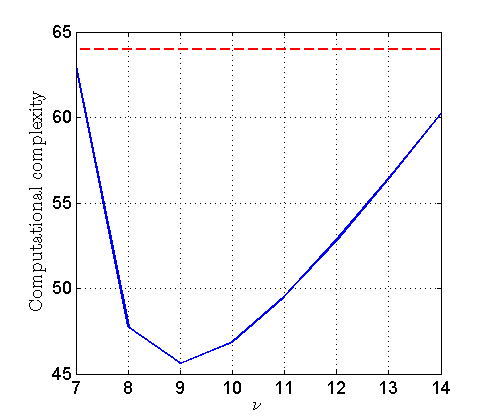### Summary

• The overlap-add method allows us to calculate the convolution of very long sequences.
• The overlap-add method breaks a long sequence, $$x(n)$$, into signals of shorter length and calculates the convolution of each block independently. To arrive at the final result, we need to apply an appropriate time shift to the convolution of the blocks and add them together.
• Analyzing the computational complexity of the DFT-based method, we observe that there is an optimum value for the length of the input blocks.

## Supporting Information

#### The Discrete Fourier Transform: